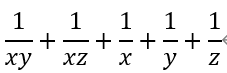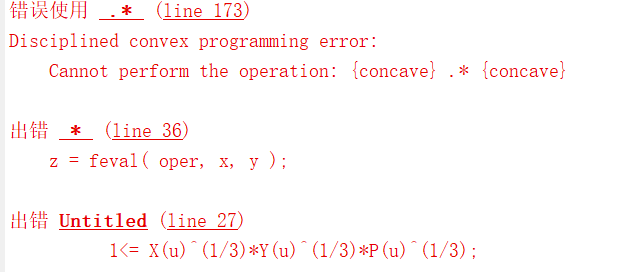# How to express the multiplication of two convex functionsHi, I want to use CVX to represent the formula in the figure above in the objective function (xyz is positive), what should I do, CVX does not seem to support multiplying two convex functions?

Assuming all variables must be nonnegative, then

(x*y*t)^{1/3} \geq 1

implies

t \geq \frac{1}{x*y}

Therefore, you can use the geometric mean to build what you want.

Alternatively, you can use prod_inv, which implements @Erling’s geometric mean formulation “under the hood”.

The built-in function inv_pos is the same as prod_inv applied to a vector consisting of one element. It providew an additional option for the single variable terms.

Just to be clear, these formulations require x,y,z all > 0, although that need not be explicitly included in the CVX code, because that will be enforced by prod_inv, inv_pos, and geo_mean.

Actually, in my problem where the variable xyz has a range of (0,1), it seems that the above inequality does not hold.

Based on the values of the variables (they range from 0 to 1), I cannot introduce the t variable. As far as I know, I can use inv_pos (x) to represent 1/x, but I can’t represent 1/ (xy).

Range of )0,1) is fine. The only requirement for use of the offered solutions is nonnegatvity of all the variables.

I tried P (u) instead of 1/[X(u)Y(u)], Q (u) instead of 1/[X(u) Z(u)], and[X(u)*Y(u)*P (u) ]^(1/3)=> 1, [X(u)*Y(u)*Q (u) ]^(1/3) => 1 are the constraints. But the results are still wrong.

U=10;

cvx_begin quiet
% cvx_solver mosek
variables X(U) Y(U) Z(U) P(U) Q(U);
Obj = 0;
for u =1:U
Obj = Obj + P(u)+Q(u)+inv_pos(X(u))+inv_pos(Y(u))+inv_pos(Z(u));
end

minimize Obj;
subject to
for u = 1:U
0 < X(u)<=1;
end

for u = 1:U
0 < Y(u)<=1;
end

for u = 1:U
0 < Z(u)<=1;
end

for u = 1:U
1<= X(u)^(1/3)*Y(u)^(1/3)*P(u)^(1/3);
end

for u = 1:U
1<= X(u)^(1/3)*Y(u)^(1/3)*Q(u)^(1/3);
end


cvx_endUse one of the formulations provided (geometric_mean or prod_inv). There will be no multiplications in the arguments of either formulation.